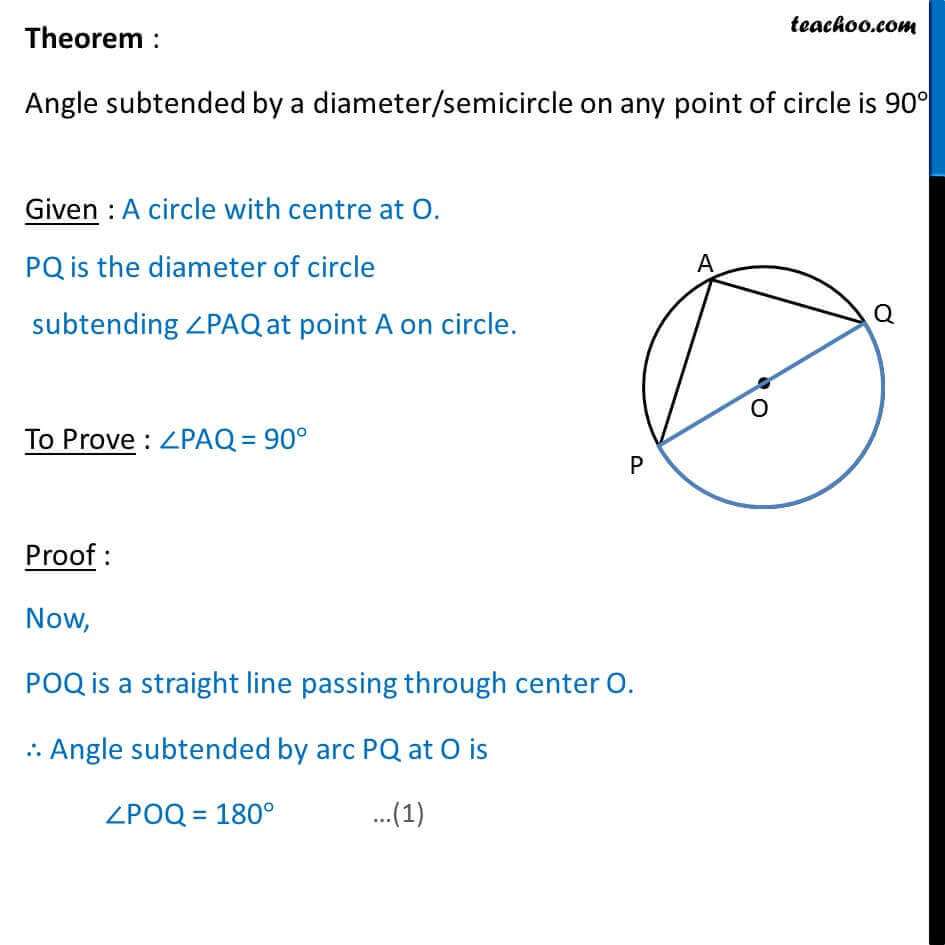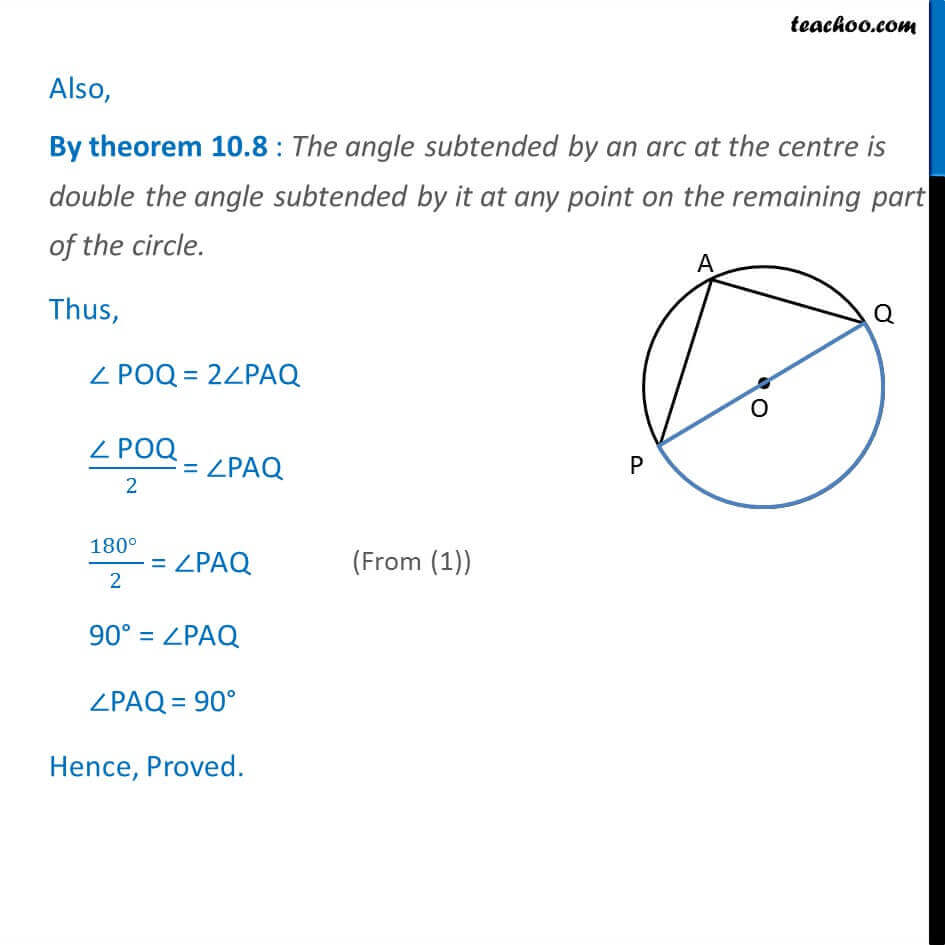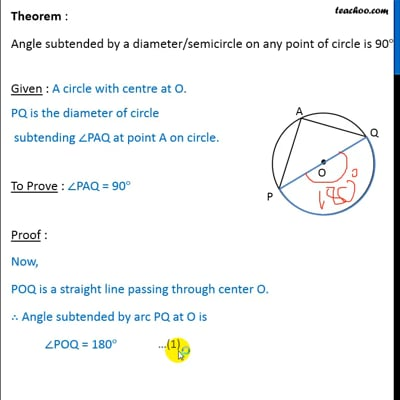Theorems

Chapter 10 Class 9 Circles
Serial order wiseThis video is only available for Teachoo black users

Introducing your new favourite teacher - Teachoo Black, at only ₹83 per month

### Transcript

Theorem : Angle subtended by a diameter/semicircle on any point of circle is 90 right angle Given : A circle with centre at 0. PQ is the diameter of circle subtending PAQ at point A on circle. To Prove : PAQ = 90 Proof : Now, POQ is a straight line passing through center O. Angle subtended by arc PQ at O is POQ = 180 Also, By theorem 10.8 : The angle subtended by an arc at the centre is double the angle subtended by it at any point on the remaining part of the circle. Thus, POQ = 2 PAQ POQ/2 = PAQ 180 = PAQ 90 = PAQ PAQ = 90 Hence, Proved.